# The length 6

The length of 12 pipes is 10 1/2 meters.
(1) find the length of one pipe
(2) also find the length of 7 pipes

a =  0.875 m
b =  6.125 m

### Step-by-step explanation: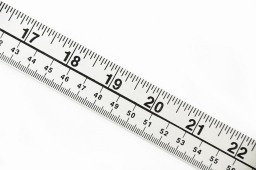Did you find an error or inaccuracy? Feel free to write us. Thank you!Tips to related online calculators
Need help to calculate sum, simplify or multiply fractions? Try our fraction calculator.
Do you want to convert length units?

## Related math problems and questions:

• Eight pipes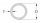Eight pipes are each 2¼m long . what is the total length of the eight pipes?
• A PVC pipeA PVC pipe 12 feet long is to be cut into 1 1/2 foot pieces.  How many pieces can a plumber get out of this length pipe?
• Two numbers and its productThe product of two numbers are 2/3. If on of them is 1/10, what is the other?
• Equivalent fractions 2Write the equivalent multiplication expression. 2 1/6÷3/4
• Divide 11Divide the product of 4 and 5/8 by 1 1/2. Write your answer as a mixed number.
• Eight 9Eight pipes are 2¼ meter long . what is the total length of the eight pipes?
• Cutting pipeA 10⅔ inch long pipe is cut into 8 equal pieces. How long is each piece?
• Penny coinsOne 2p coin is 1/8 inch thick. A pile of 2p is 1 1/2 inch high. How many coin are there in the pile
• The product 2The product of two functions is 10. If one of them is 2 1/3, find the other one.
• The fence 2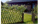A certain type of fencing sells for \$9 a yard. How much will 10 2/3 yards of fencing cost?
• Between two mixed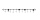What is the rational number between 2 1/4 and 2 4/5?
• Wood 11Father has 12 1/5 meters long wood. Then I cut the wood into two pieces. One part is 7 3/5 meters long. Calculate the length of the other wood?
• Fractions and mixed numerals(a) Convert the following mixed numbers to improper fractions. i. 3 5/8 ii. 7 7/6 (b) Convert the following improper fraction to mixed number. i. 13/4 ii. 78/5 (c) Simplify these fractions to their lowest terms. i. 36/42 ii. 27/45 2. evaluate following exAdd two mixed fractions: 2 4/6 + 1 3/6With what number should be divided mixed number 2 3/4 to get 11/12?Sort fractions z1 = (6)/(11); z2 = (10)/(21); z3 = (19)/(22) by its size. Result write as three serial numbers 1,2,3.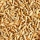The bird feeder holds 1 and 1/2 cups of birdseed. Reagan is using a scoop that holds 1/8 of a cup. How many scoops will she need to fill the bird feeder?